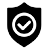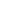#### WhatsApp Quick Chat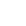#### Offers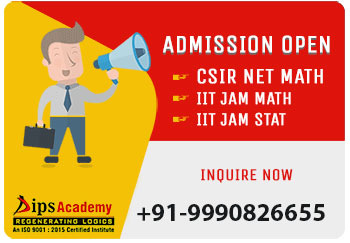##### News & Events# IIT JAM Syllabus

#### IIT JAM Mathematics & Mathematical Statistics Syllabus

Mathematics (MA)

Real Analysis
Sequences and Series of Real Numbers: Convergence of sequences, bounded and monotone sequences, Cauchy sequences, Bolzano-Weierstrass theorem, absolute convergence, tests of convergence for series – comparison test, ratio test, root test; Power series (of one real variable), radius and interval of convergence, term-wise differentiation and integration of power series. Functions of One Real Variable: L imit, continuity, intermediate value property, differentiation, Rolle’s Theorem, mean value theorem, L'Hospital rule, Taylor's theorem, Taylor’s series, maxima and minima, Riemann integration (definite integrals and their properties), fundamental theorem of calculus.

Multivariable Calculus and Differential Equations
Functions of Two or Three Real Variables: : Limit, continuity, partial derivatives, total derivative, maxima and minima. Integral Calculus: Double and triple integrals, change of order of integration, calculating surface areas and volumes using double integrals, calculating volumes using triple integrals. Differential Equations: Bernoulli’s equation, exact differential equations, integrating factors, orthogonal trajectories, homogeneous differential equations, method of separation of variables, linear differential equations of second order with constant coefficients, method of variation of parameters, Cauchy-Euler equation.

Linear Algebra and Algebra:
Matrices: Systems of linear equations, rank, nullity, rank-nullity theorem, inverse, determinant, eigenvalues, eigenvectors. Finite Dimensional Vector Spaces: Linear independence of vectors, basis, dimension, linear transformations, matrix representation, range space, null space, rank-nullity theorem. Groups: Cyclic groups, abelian groups, non-abelian groups, permutation groups, normal subgroups, quotient groups, Lagrange's theorem for finite groups, group homomorphisms.

MATHEMATICAL STATISTICS (MS)

The Mathematical Statistics (MS) Test Paper comprises following topics of Mathematics (about 30% weight) and Statistics (about 70% weight).

Mathematics
Sequences and Series of real numbers: Sequences of real numbers, their convergence, and limits. Cauchy sequences and their convergence. Monotonic sequences and their limits. Limits of standard sequences. Infinite series and its convergence, and divergence. Convergence of series with non-negative terms. Tests for convergence and divergence of a series. Comparison test, limit comparison test, D’Alembert’s ratio test, Cauchy’s 𝑛 𝑡ℎ root test, Cauchy’s condensation test and integral test. Absolute convergence of series. Leibnitz’s test for the convergence of alternating series. Conditional convergence. Convergence of power series and radius of convergence. Differential Calculus of one and two real variables: Limits of functions of one real variable. Continuity and differentiability of functions of one real variable. Properties of continuous and differentiable functions of one real variable. Rolle's theorem and Lagrange's mean value theorems. Higher order derivatives, Lebnitz's rule and its applications. Taylor's theorem with Lagrange's and Cauchy's form of remainders. Taylor's and Maclaurin's series of standard functions. Indeterminate forms and L' Hospital's rule. Maxima and minima of functions of one real variable, critical points, local maxima and minima, global maxima and minima, and point of inflection. Limits of functions of two real variables. Continuity and differentiability of functions of two real variables. Properties of continuous and differentiable functions of two real variables. Partial differentiation and total differentiation. Lebnitz's rule for successive differentiation. Maxima and minima of functions of two real variables. Critical points, Hessian matrix, and saddle points. Constrained optimization techniques (with Lagrange multiplier). Integral Calculus: Fundamental theorems of integral calculus (single integral). Lebnitz's rule and its applications. Differentiation under integral sign. Improper integrals. Beta and Gamma integrals: properties and relationship between them. Double integrals. Change of order of integration. Transformation of variables. Applications of definite integrals. Arc lengths, areas and volumes. Matrices and Determinants: Vector spaces with real field. Subspaces and sum of subspaces. Span of a set. Linear dependence and independence. Dimension and basis. Algebra of matrices. Standard matrices (Symmetric and Skew Symmetric matrices, Hermitian and Skew Hermitian matrices, Orthogonal and Unitary matrices, Idempotent and Nilpotent matrices). Definition, properties and applications of determinants. Evaluation of determinants using transformations. Determinant of product of matrices. Singular and nonsingular matrices and their properties. Trace of a matrix. Adjoint and inverse of a matrix and related properties. Rank of a matrix, row-rank, column-rank, standard theorems on ranks, rank of the sum and the product of two matrices. Row reduction and echelon forms. Partitioning of matrices and simple properties. Consistent and inconsistent system of linear equations. Properties of solutions of system of linear equations. Use of determinants in solution to the system of linear equations. Cramer’s rule. Characteristic roots and Characteristic vectors. Properties of characteristic roots and vectors. Cayley Hamilton theorem.

Statistics
Probability: Random Experiments. Sample Space and Algebra of Events (Event space). Relative frequency and Axiomatic definitions of probability. Properties of probability function. Addition theorem of probability function (inclusion exclusion principle). Geometric probability. Boole's and Bonferroni's inequalities. Conditional probability and Multiplication rule. Theorem of total probability and Bayes’ theorem. Pairwise and mutual independence of events. Univariate Distributions:Definition of random variables. Cumulative distribution function (c.d.f.) of a random variable. Discrete and Continuous random variables. Probability mass function (p.m.f.) and Probability density function (p.d.f.) of a random variable. Distribution (c.d.f., p.m.f., p.d.f.) of a function of a random variable using transformation of variable and Jacobian method. Mathematical expectation and moments. Mean, Median, Mode, Variance, Standard deviation, Coefficient of variation, Quantiles, Quartiles, Coefficient of Variation, and measures of Skewness and Kurtosis of a probability distribution. Moment generating function (m.g.f.), its properties and uniqueness. Markov and Chebyshev inequalities and their applications. Standard Univariate Distributions: Degenerate, Bernoulli, Binomial, Negative binomial, Geometric, Poisson, Hypergeometric, Uniform, Exponential, Double exponential, Gamma, Beta (of first and second type), Normal and Cauchy distributions, their properties, interrelations, and limiting (approximation) cases. Multivariate Distributions: Definition of random vectors. Joint and marginal c.d.f.s of a random vector. Discrete and continuous type random vectors. Joint and marginal p.m.f., joint and marginal p.d.f.. Conditional c.d.f., conditional p.m.f. and conditional p.d.f.. Independence of random variables. Distribution of functions of random vectors using transformation of variables and Jacobian method. Mathematical expectation of functions of random vectors. Joint moments, Covariance and Correlation. Joint moment generating function and its properties. Uniqueness of joint m.g.f. and its applications. Conditional moments, conditional expectations and conditional variance. Additive properties of Binomial, Poisson, Negative Binomial, Gamma and Normal Distributions using their m.g.f.. Standard Multivariate Distributions: Multinomial distribution as a generalization of binomial distribution and its properties (moments, correlation, marginal distributions, additive property). Bivariate normal distribution, its marginal and conditional distributions and related properties. Limit Theorems: Convergence in probability, convergence in distribution and their inter relations. Weak law of large numbers and Central Limit Theorem (i.i.d. case) and their applications. Sampling Distributions: Definitions of random sample, parameter and statistic. Sampling distribution of a statistic. Order Statistics: Definition and distribution of the 𝑟 𝑡ℎ order statistic (d.f. and p.d.f. for i.i.d. case for continuous distributions). Distribution (c.d.f., p.m.f., p.d.f.) of smallest and largest order statistics (i.i.d. case for discrete as well as continuous distributions). Central Chi-square distribution: Definition and derivation of p.d.f. of central 𝜒2 distribution with 𝑛 degrees of freedom (d.f.) using m.g.f.. Properties of central 𝜒2 distribution, additive property and limiting form of central 𝜒2 distribution. Central Student's 𝒕-distribution: Definition and derivation of p.d.f. of Central Student's 𝑡-distribution with 𝑛 d.f., Properties and limiting form of central 𝑡-distribution. Snedecor's Central 𝑭-distribution: Definition and derivation of p.d.f. of Snedecor's Central 𝐹-distribution with (𝑚, 𝑛) d.f.. Properties of Central 𝐹-distribution, distribution of the reciprocal of 𝐹- distribution. Relationship between 𝑡, 𝐹 and 𝜒2 distributions. Estimation: Unbiasedness. Sufficiency of a statistic. Factorization theorem. Complete statistic. Consistency and relative efficiency of estimators. Uniformly Minimum variance unbiased estimator (UMVUE). RaoBlackwell and Lehmann-Scheffe theorems and their applications. Cramer-Rao inequality and UMVUEs. Methods of Estimation: Method of moments, method of maximum likelihood, invariance of maximum likelihood estimators. Least squares estimation and its applications in simple linear regression models. Confidence intervals and confidence coefficient. Confidence intervals for the parameters of univariate normal, two independent normal, and exponential distributions. Testing of Hypotheses: Null and alternative hypotheses (simple and composite), Type-I and Type-II errors. Critical region. Level of significance, size and power of a test, p-value. Most powerful critical regions and most powerful (MP) tests. Uniformly most powerful (UMP) tests. Neyman Pearson Lemma (without proof) and its applications to construction of MP and UMP tests for parameter of single parameter parametric families. Likelihood ratio tests for parameters of univariate normal distribution.

## Offers## News & Events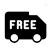##### Free Delivery